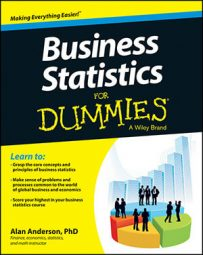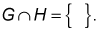An event is one possible outcome of a random experiment. Events may sometimes be related to each other. Two key ways in which events may be related are known as mutually exclusive and independent.

## How to Identify Mutually Exclusive Events

Two events are said to be mutually exclusive if they can't both happen at the same time. Here are two events that are mutually exclusive:

A = The roll of a die is odd.

B = The roll of a die is even.

Clearly, the roll of a die must result in a number that is either odd or even; it can't be both. Therefore, events A and B are mutually exclusive.

As another example, in a coin-flipping experiment, suppose that two events are defined:

G = Two heads turn up.

H = Two tails turn up.

It's impossible for both two heads to turn up and two tails to turn up. This means that G and H are mutually exclusive. This result can be demonstrated using sets as follows:

G = {HH} and H = {TT}. These events have no elements in common; their intersection is the empty setThe probability of the empty set is zero; therefore, the event that both G and H occur is impossible. This means that G and H are mutually exclusive.

## How to Identify Independent Events

Two events A and B are said to be independent if the outcome of event A doesn't affect the outcome of event B and vice versa. For example, suppose that based on the coin-flipping experiment, event A is defined as the event that the first flip is a head, and event B is defined as the event that the second flip is a head. In other words:

A = {HH, HT}

B = {HH, TH}

Because the outcome of the first flip has no influence over the outcome of the second flip, events A and B are independent events.

Note that A and B are not mutually exclusive; both A and B can occur.